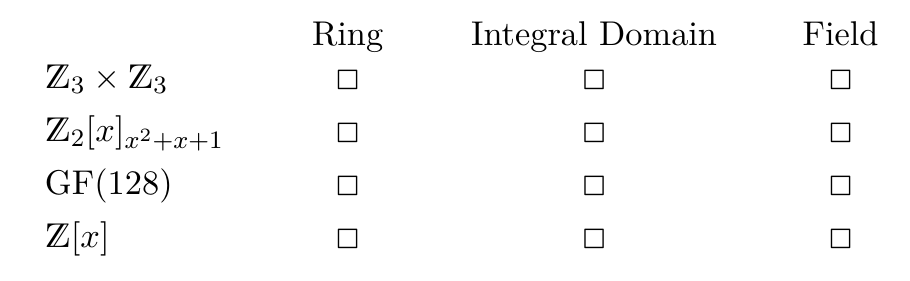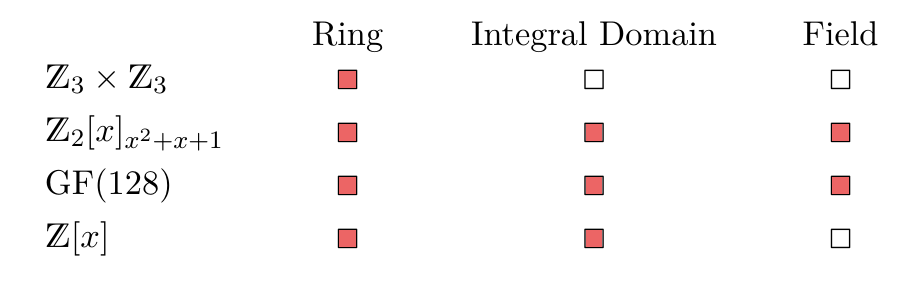Today, we’ll investigate the following exercise:

Decide whether the given algebra is a ring, a field, or an integral domain (check all that apply). (FS18, 4b)Let us examine those algebras one by one.

$\mathbb{Z}_3 \times \mathbb{Z}_3$ is a direct product of two rings (because all $\mathbb{Z}_m$ are commutative rings). The direct product of two rings is a ring. (You could show this by checking that all the axioms hold.) $\mathbb{Z}_3 \times \mathbb{Z}_3$ isn’t an integral domain though - the counterexample $(0,1) * (1, 0) = (0,0)$ shows that there exists a zerodivisor. Since a field is always an integral domain (Theorem 5.24), this algebra cannot be a field as it isn’t an integral domain.

$\mathbb{Z}_2 [x]_{x^2+x+1}$ is a field (see this earlier blog post to find out how to prove that). That also makes it a ring, since a field is merely a “special case” of a ring and an integral domain as stated by Theorem 5.24.

$\text{GF}(128)$ certainly is a field (GF stands for Galois-Field after all). Therefore it is also a ring and an integral domain (same reasoning as above).

Finally, $\mathbb{Z}[x]$ is a ring since $\mathbb{Z}$ is a commutative ring and for any ring $R$, $R[x]$ is also a ring (Theorem 5.21). Additionally, the same reasoning applies for $\mathbb{Z}[x]$ being an integral domain: $Z$ is an integral domain, and by Lemma 5.22 i), if $D$ is an integral domain, then so is $D[x]$. It is not a field though, since e.g. $5x$ does not have an inverse and is therefore not a unit.